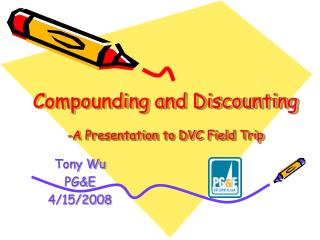DownloadDownload PresentationCompounding and Discounting -A Presentation to DVC Field Trip

# Compounding and Discounting -A Presentation to DVC Field Trip

Télécharger la présentation## Compounding and Discounting -A Presentation to DVC Field Trip

- - - - - - - - - - - - - - - - - - - - - - - - - - - E N D - - - - - - - - - - - - - - - - - - - - - - - - - - -
##### Presentation Transcript

1. Compounding and Discounting-A Presentation to DVC Field Trip Tony Wu PG&E 4/15/2008

2. Compounding and Discounting are used to calculate: • How much is one dollar now worth at t years later? • How much is one dollar at t years later worth now?

3. Interest • The time value of money • In your bank account, \$1 now (the present value) becomes \$1.06 one year later (the future value) • The interest is \$0.06 • The interest rate is \$0.06/\$1 = 6% per year • When do you pay interests? • Student loans, Credit Cards, Mortgage • When do you receive interests? • Saving accounts

4. Compound Interest • Present Value, V0 • Interest rate, r per year, calculate the interest once per year • One year later • V1=V0 (1+r) • Two years later • V2=V1 (1+r) = V0 (1+r)^2 • N years later • VN = V0 (1+r)^N

5. Compounding at Various Intervals • Present Value, V0; Nominal rate, r per year • One and two years later • If compounding quarterly • V1 = V0 [ 1 + (r/4) ]^4 • V2 = V0 [ 1 + (r/4) ]^8 • If compounding monthly • V1 = V0 [ 1 + (r/12) ]^12 • V2 = V0 [ 1 + (r/12) ]^24 • If compounding daily • V1 = V0 [ 1 + (r/365) ]^365 • V2 = V0 [ 1 + (r/365) ]^730

6. Effective interest rate • Nominal rate, r per year • Compounding m times per year • Effective rate, r’ per year • 1 + r’ = [ 1 + (r / m) ] ^ m • Example, the Credit Card APR (annual percentage rate) is a nominal rate and compounds monthly • If the APR is 15% • Then the effective APR is • 1 + r’ = [ 1 + (15% / 12) ] ^ 12 • r’ = 16.1%

7. Continuous Compounding • Nominal rate, r per year • Compounding at infinite small time intervals • Effective rate, r’ per year

8. Continuous Compounding • Present Value, V0 • Nominal rate, r per year • One year later • V1 = V0 exp (r) • Two year later • V2 = V1 exp (r) = V0 exp (2r) • t year later (t is a real number) • Vt = V0 exp (rt)

9. Discounting • How much is Vt dollar at t years later worth now? • Nominal rate, r per year • Discount factor, Dt, is the factor by which the value at t years later must be multiplied to obtain an equivalent present value • Compounding m periods per year • V0 = Vt [ 1 + (r / m) ] ^ (- m t) = Dt Vt • Dt = [ 1 + (r / m) ] ^ (- m t) • Continuous Compounding • V0 = Vt exp (- r t) = Dt Vt • Dt = exp (- r t)

10. Compounding and Discounting with variable interest rate • Present Value, V0 • Variable interest rate, r(u) at time u • Continuous compounding • Value at time t, Vt • Discount Factor

11. Application of Discounting • Present Value of a future cash flow • At time t, t=1,2, … N; Payment Vt; Discount factor Dt; then the PV is • Could be used to compare two projects

12. Unit PV and levelization calculation • At time t, t=1,2, … N; Payment Vt per unit; Volume at t, Ut unit; Discount factor Dt; • The Unit PV is • The levelized PV is

13. Either PV is validated, implicating different views of the value of project in the future • A Project receives \$1 million 1 year later, D1=0.75, and \$3 million 2 years later, D2 = 0.5 • If we use the unit PV method, then V0U=(1X0.75+3X0.5)/2=1.125 million • It means that the average unit present value is 1.125 million • If we use the levelized PV method, then V0L=(1X0.75+3X0.5)/(0.75+0.5) = 1.8 million • It is equivalent to receive a flat future cash flow • V1 = 1.8 million • V2 = 1.8 million